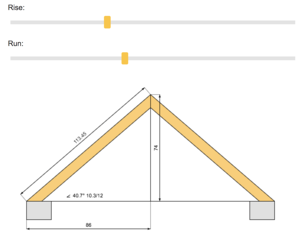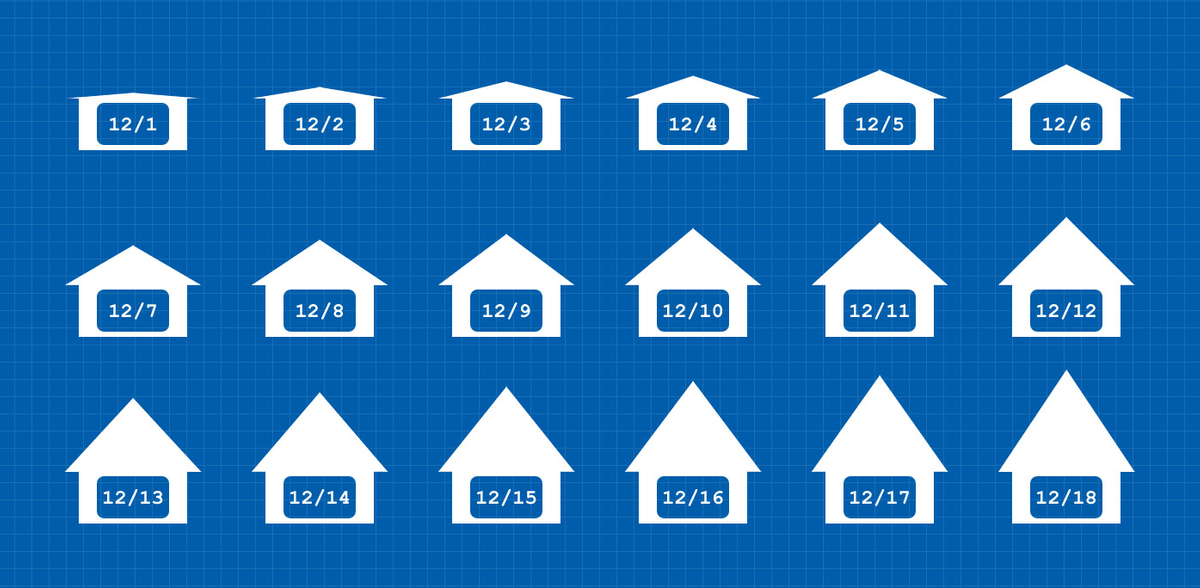4 Great Roof Pitch Calculators to Determine Pitch, Angle, and More | Free Roofing Estimates & Quotes Find a Local Roofing Contractor Roofr

## 4 Great Roof Pitch Calculators to Determine Pitch, Angle, and More

Some of the most important things to know about your roof are its measurements, since roofing materials and cost depend on several key roof measurements, including pitch and angle. You can always calculate these numbers with a tape measure and a calculator or smartphone, but this leaves too much room for mistakes. Instead, let’s cover some online roof pitch calculators that will make this process easier and yield more accurate results.

## #1 Blocklayer.com

Blocklayer.com is a classic “old school website” in design style, but it provides a perfect solution for quickly converting between pitch, angle, and grade. A slider lets you adjust the angle, and the other measurements instantly adjust as you drag the tab on the slider. The website even shows you a right triangle that updates with the measurements, so you can easily understand the geometric relationships that are crucial to roof pitch calculations and other architectural measurements. Feel like you’re back in high school yet?## #2 Omni Calculator

Omni Calculator has a beautifully designed roof pitch calculator. Simply enter the rise and run of your roof, and choose from several units of measurement, including feet, inches, and meters.

After you type in the rise and run, the calculator instantly tells you the rafter length, grade, and roof pitch in x:12. For reference, roof pitch refers to the distance your roof goes up for every 12 feet in. For example, 4:12 or 8:12 pitch.

The calculator will also tell you the roof pitch angle in degrees, radians, and more. Once you get the results from Omni Calculator, you can send them to yourself or save a unique link to your specific results.

## #3 MyCarpentry.com

Another great roof pitch calculator comes from MyCarpentry.com. You enter the rise and run, but you can only do so in inches. The slope calculator will also determine angle, rafter length, and pitch.

On its calculator page, MyCarpentry.com also includes roofing definitions and diagrams to help you better understand what to measure and what the calculator results mean.## #4 MyRooff.comFor the roof pitch calculator on MyRooff.com, you need to know two of these three measure ments: rise, run, and rafter length. Your measurements must be in inches. After you enter your two measurements, the calculator will determine pitch, angle, grade, as well as the third measurement you did not enter.

The calculator has sliders you can use to adjust the measurements and instantly see updated numbers, as well as a handy, dynamic roof diagram that shows your measurements and calculations on it.

## Bonus! #5 Your amazing, internal, fat-burning calculator

Even if you choose to use an online roof pitch calculator, understanding how the calculations work is part of the beauty in being human. While machines are highly efficient at making repeated, near-instant mathematical calculations, the human brain is infinitely better at  creative problem-solving and pattern discovery. Review the common roof measurements below to help your amazing brain make sense of how this all works.### Pitch

The pitch, or slope, of a roof is determined by its vertical rise in inches for every 12 inches of horizontal run. You will need to know the pitch of your roof to calculate the total roof area. This information is essential to determine how much material is necessary to cover the area.

Pitch can also help you identify which roof covering you currently have installed. Each type of roof covering is suitable for a specific range of pitches, which tie into factors like rain and snow runoff, as well as ice buildup, and how difficult it will be to walk on your roof.

To calculate pitch, measure the height of the tallest part of the roof (the ridge), and measure from the outer edge of the bottom of the roof to directly under the ridge (the run). Then, simply divide the rise by the run.

This ratio can be written in a couple of formats and is best expressed in inches per foot. The most common of these formats are x:12 or x/12, which indicates the number of inches of rise for every 12 inches of run. For example, a roof that rises four inches for every 12-inch run has a 4/12 or 4:12 pitch.

### AngleA roof’s angle expresses the same information as its pitch, however angles are represented in degrees instead of two-value ratios. The degree measures the angle where your house and the edge of the roof meet.

Knowing the angle comes in handy for activities like cutting roofing materials with a saw. Saws are usually calibrated in degrees, so ratios won’t compute.

You can calculate the angle by taking the grade and multiplying it by an arctan. The equation looks like this: angle = arctan (grade). You might want to use an arctan calculator for this. The equation will give you the angle in radians. But, you can convert the radians to degrees. The equation to convert radians to degrees is: degrees = radians x 180° / 𝝅.

Let’s use the grade from the previous example, 33- percent. The equation will look like this: angle = arctan (0.3333). The result is 0.32 radians. Now, you can convert the radians into degrees. Use the conversion equation: degrees = 0.32 x 180° / 𝝅. In this case, 0.32 radians equals 18.43°.

It might be easiest to calculate the roof angle using a roof pitch calculator. Or, if you know your roof’s pitch, you can easily convert to the angle by using a conversion table.